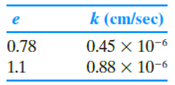Chapter 7, Problem 7.18PPrinciples of Geotechnical Enginee...

9th Edition
Braja M. Das + 1 other
ISBN: 9781305970939

Solutions

Chapter
SectionPrinciples of Geotechnical Enginee...

9th Edition
Braja M. Das + 1 other
ISBN: 9781305970939
Textbook Problem

For a normally consolidated clay, the following values are given.Estimate k at a void ratio of 0.97. Use Eq. (7.38).

To determine

Find the hydraulic conductivity k3 of the sand at void ratio of 0.97.

Explanation

Given information:

The hydraulic conductivity (k1) at void ratio (e1) of 0.78 is 0.45×106cm/sec.

The hydraulic conductivity (k2) at void ratio (e2) of 1.1 is 0.88×106cm/sec.

The given void ratio (e3) is 0.97.

Calculation:

Determine the constant value n using the relation.

k1k2=(e1n1+e1)(1+e2e2n)=(1+e21+e1)(e1e2)n (1)

Substitute 0.45×106cm/sec for k1, 0.88×106cm/sec for k2, 0.78 for e1, and 1.1 for e2 in Equation (1).

0.45×1060.88×106=(1+1.11+0.78)(0.781.1)n0.5111.179=0.709n0

Still sussing out bartleby?

Check out a sample textbook solution.

See a sample solution

The Solution to Your Study Problems

Bartleby provides explanations to thousands of textbook problems written by our experts, many with advanced degrees!

Get Started